# Solving Equations with Wolfram|Alpha

January 14, 2010 —Comments Off

Need a tutor for solving equations? Solving equations is just one of hundreds of mathematical tasks that can be done using Wolfram|Alpha. Wolfram|Alpha can solve equations from middle school level all the way through college level and beyond. So next time you are stumped on an equation, consult Wolfram|Alpha for a little help.

Let’s start with the simpler stuff. Wolfram|Alpha can easily solve linear and quadratic equations, and even allows you to view a step-by-step solution of each problem.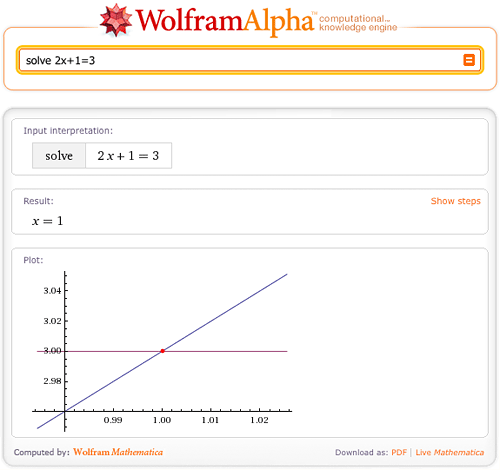What if the roots of the equation are complex? No worries; Wolfram|Alpha has no trouble solving equations over the complex plane.Wolfram|Alpha can also solve cubic and quartic equations in terms of radicals.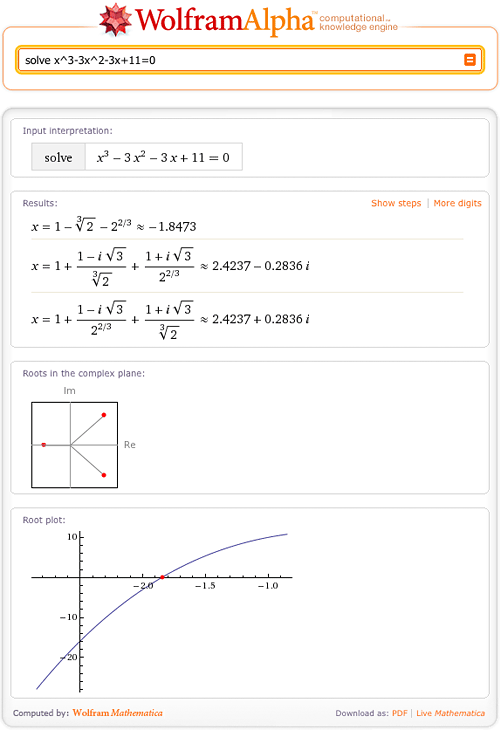Of course, some solutions are too large or cannot be represented in terms of radicals; Wolfram|Alpha will then return numerical solutions with a “More digits” button.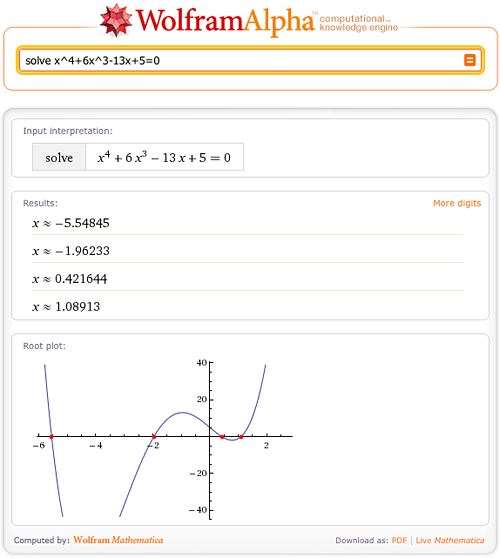Conveniently, Wolfram|Alpha not only solves polynomial equations but also equations involving trigonometric, hyperbolic, or even special functions, as in the following example.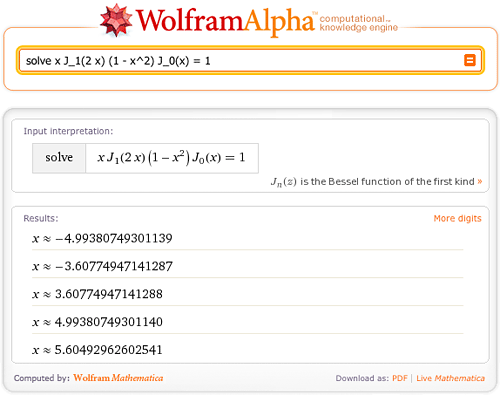Do you want to solve an equation over the reals? Just tell Wolfram|Alpha to restrict the domain.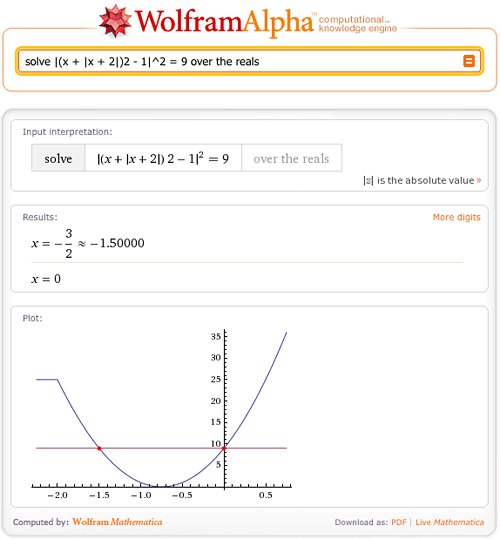Wolfram|Alpha can also solve systems of linear and nonlinear equations.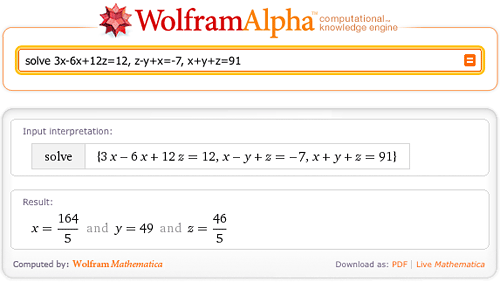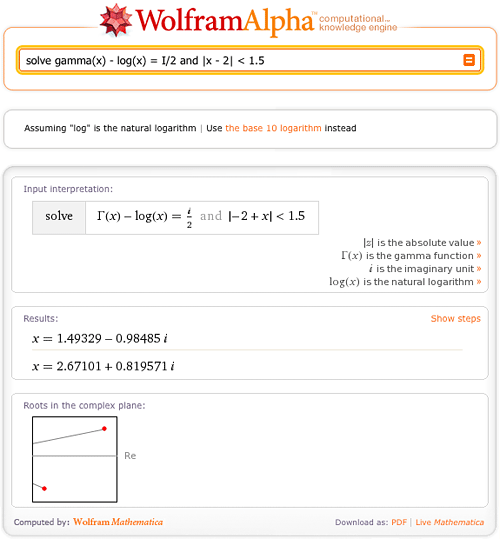In the near future, it will be possible to see step-by-step solutions for systems of linear equations

Let’s take it one step further. Do you need to solve a system of polynomial congruences? Wolfram|Alpha is not stumped!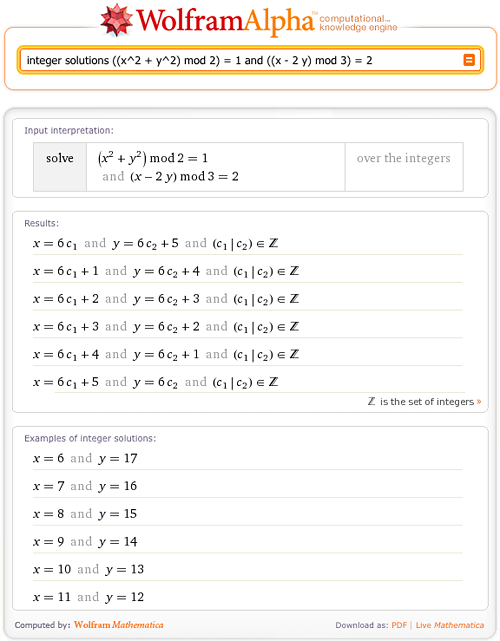Are you working with recurrence relations? Wolfram|Alpha can solve recurrence equations in seconds.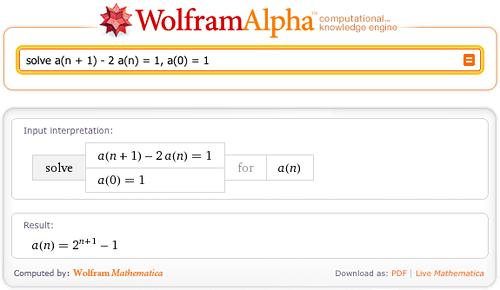For more, check out the Examples pages. The underlying technology used here are the Mathematica functions FindInstance, Solve, RSolve, FindRoot, NSolve and Reduce.

Remember that these are just a few of the ways that Wolfram|Alpha can solve equations—try some of your own math problems and explore other equation types. In a future blog post, we’ll show you how Wolfram|Alpha can also solve inequalities and systems of inequalities.

I noticed that it can show steps. I was thrilled when I typed in 1/(1-x^2) as it not only split it into partial fractions, but it revealed in the relationship that the above has with tanh^-1(x) upon integration.

This makes it an even better reference and educational resource, but I was wondering if there was any software available for Mathematica to enable similar functionality.

Posted by gm January 14, 2010 at 6:09 pm

The solutions to fourth degree polynomial equations can always be expressed in terms of radicals. The solutions to the quartic in the given example all were less than 6 in absolute value. “Of course, some solutions are too large or cannot be represented in terms of radicals”?!

Perhaps Wolfram|Alpha does not want to display the exact solutions because they are messy, but those solutions can be expressed in terms of radicals and are not large.

Posted by Bruce Yoshiwara January 15, 2010 at 12:00 am

It is sad that wolfram doesn’t have a Polish translation.
Unfortunately, someone bought the pl domain.

Posted by atominium January 18, 2010 at 9:50 am

I used wolfram alpha to teach my student about solving equations,I put some examples in my blog, htttp://abdulkarim.wordpress.com

Posted by Abdul Karim February 6, 2010 at 7:52 pm

Still has a long way to go. I wanted to solve (1 + 1.6*10^-19*x/(1.38*10^-23 * 300))*exp(1.6*10^-19*x/(1.38*10^-23 * 300)) = 35.68/30*10^-9 and it returned x = 0.025875 W_n((3568000000 e)/3)-0.025875 and n element Z, as the result. Strange. I did same thing with Matlab fsolve and got 0.464 which is the solution I was looking for. Alpha still has a long way to go. I didn’t think I would still need MATLAB for little things like this…..

Posted by Ob February 9, 2010 at 4:47 pm

I cannot get Wolfram|alpha to solve a system (linear or otherwise) larger than 3 by 3. Has anyone been able to do this? Yes, I have read all of the tutorials and I am familiar with the RREF command, but I’d just like to enter in the equations and see the solution. Imagine telling someone to get the solution to a 5 by 5 system, but having to teach them basic matrix operations first. That’s kind of annoying.

All the previous notwithstanding, I very much like Wolfram|alpha and will continue to use it in the future. I just wish solving a “larger” system was easily done.

Posted by sven February 10, 2010 at 1:59 am

Can Wolfram solve linear inequalities?

Posted by Sean January 30, 2011 at 4:50 pm

Posted by janine February 15, 2011 at 1:35 pm

can wolfram alpha solve radicals like finding the 5th root of -32?

Posted by brandon February 23, 2011 at 1:24 pm

Can I solve a trig equation for restricted values of theta

eg solve tan(th +pi/4) = 1 – 2tan(th) for theta from 0 to pi/2

and can I do that in degrees?

Posted by Colleen Young March 13, 2011 at 3:34 am

Hello, I was wondering if Wolfram can solve system problems, such as:

4x + 5y – z = -38
3x – 4y = -3
4x + 3y = -29

Thanks!

Posted by Rachel March 22, 2011 at 12:28 pm

Hi I’m trying to use wolfram a large system of equations entirely symbolically. Wolfram can’t seem to handle the equations. The following is what I entered, an example of only the first two equations:
Paf= (Taf/Ta1)^Cp/R, Paf= Pa1*Va1*Taf/(Ta1*(Va1+Vb1-Vbf))

Posted by Annie March 28, 2011 at 11:30 pm

Would you, please, teach us how to get answers assuming all functions in equations are over real numbers? The point is that W|A practically always knows on complex numbers. That is correct, of course.

However many elementary problems are restricted to a field of real numbers (for those who do not know “i”).

So, tryng to solve equations with radical one gets solutions where some terms of eq. become complex. The similar story is with trigonometry. Very often one gets answers like sin^(-1) 4 etc. That is fine for advanced in math people, but can be treated as a “mistake” in an elementary school…

The commands like Reduce[…, Real] doesn’t provide that restriction.

Posted by gorod March 31, 2011 at 12:03 am

hi there
i have a problem and i want to solve a set of equations altogether but i can’t.
i appreciate if yo can help me.
thank you
we have 12 equations and 12 unknowns.
here is the equations:

f1 = y_CO + y_CO2 + y_H2O + y_O2 + y_NO + y_H2 + y_N2 == 1
f2 = 1.641*10^-006*T^2 – 0.09051*T – 111.1 + R*T*Log[y_CO] +
lambda_C + lambda_O == 0
f3 = 8.221*10^-022*T^6 – 1.865*10^-017*T^5 + 1.676*10^-013*T^4 –
7.443*10^-010*T^3 + 2.381*10^-006*T^2 – 0.004387*T – 393.3 +
R*T*Log[y_CO2] + lambda_C + 2*lambda_O == 0
f4 = 8.058*10^-014*T^4 – 1.165*10^-009*T^3 + 6.132*10^-006*T^2 +
0.04503*T – 242.4 + R*T*Log[y_H2O] + lambda_O + 2*lambda_H == 0
f5 = R*T*Log[y_N2] + 2*lambda_N == 0
f6 = R*T*Log[y_H2] + 2*lambda_H == 0
f7 = R*T*Log[y_O2] + 2*lambda_O == 0
f8 = -0.01236*T + 89.93 + R*T*Log[y_NO] + lambda_N + lambda_O == 0
f9 = y_CO2 + y_CO – 3/sigma_n == 0
f10 = 2 y_CO2 + y_CO + y_H2O + y_NO + 2 y_O2 – 10/sigma_n == 0
f11 = 2 y_N2 + y_NO – 37.62/sigma_n == 0
f12 = 2 y_H2O + 2 y_H2 – 8/sigma_n == 0

and unknowns are y_CO, y_CO2, y_H2, y_H2O, y_N2, y_NO, y_O2, lambda_C, lambda_O, lambda_H, lambda_N, sigma_n

i am waiting for the answer
tnx again

Posted by mohammad April 17, 2011 at 11:52 pm

hello i’m interested if wolfram can solve this !

http://imageshack.us/photo/my-images/109/matxf.jpg/

if anyone has an answer i will be veryyyyy thankful…..

>my mail < majosisk@gmail.com

Posted by Marian May 24, 2011 at 10:01 am

I am able to use alpha to find the particular solution to a differential equation with initial condition like:

{f”(x) = 1, f'(0) = 0, f(0) = 1, x = 1}, i.e. f(1) = 3/2

but not for:

{f””(x)=1, f”(0)=0, f(0)=0,f”(2)=0, f(2)=0, x=1}, i.e. f(1)=5/24

Alpha produces the solution, i.e. f(x) = 1/24 x (x^3-4 x^2+8), but does not substitute x=1 like before

Is alpha able to produce the particular solution for this equation in one go? Or, alternatively, can I use the outcome of one query to feed the next? Thanks!

Posted by harry June 5, 2011 at 7:42 am

Can this solve of system of equations… like this where I have m equations, n variables and a few values that are known? situations that can be done on paper?

2x+ 3y+ Z=10
xy+sin(z)=5
y=2

Posted by RG July 30, 2011 at 10:38 am

hello,how can I solve these equations with mathematica?
3x+6y^2+z=30
5x+8y^2+z=37
7x+9y^2+z=28

Posted by mohsen January 19, 2012 at 10:01 am

Can WolframAlpha transpose an equation to make a certain variable the subject?
I have this equation >>> x=y*((1+B)/B)*(1-((D/d)^(2*B))) <<<
I need to solve for B… Any help?

Posted by Brad May 8, 2012 at 12:07 am

A comment related to the above quote: “In the near future, it will be possible to see step-by-step solutions for systems of linear equations.” As of today June 23, 2013 you still can not see the step by step solution of it solving a system of linear equations. Or if this exists please inform me how to do it.

Posted by Charlotte Dyck June 23, 2013 at 8:02 pm

can WolframAlpha convert an equation containing square roots into a polynomial one with the same solutions, e.g. sqrt((x+a+1)^2+(y+b)^2)+sqrt(1-(x-a))^2+(y-b)^2) = c?
That can be done by hand by repeated squaring; can WolframAlpha do it for me?

thanks!

Posted by QuickMath July 27, 2014 at 11:36 am

Hi, we tried to solve this equation for r which worked fine: http://www.wolframalpha.com/input/?i=solve+0+%3D+r^3+%2B+O%2F%28-2*pi%29*r+%2B+V%2Fpi+for+r&dataset=&equal=Submit

However, when we copied the data* (via copyable plaintext) and submitted it again to wolframalpha, the error was thrown: “Standard computation time exceeded…”

Can wolframalpha solve this? Actually we just need to give V and O a value and see what the results are…

* r = O/(6^(1/3) (sqrt(6) pi^(3/2) sqrt(54 pi V^2-O^3)-18 pi^2 V)^(1/3))+(sqrt(6) pi^(3/2) sqrt(54 pi V^2-O^3)-18 pi^2 V)^(1/3)/(6^(2/3) pi)

Posted by Mathematik Online lernen August 15, 2014 at 2:24 pm

Boa tarde. Estou a desenvolver uma dissertação em Matemática, onde faço uso do software Mathematica 9.0. Uso a função NSolve do seguinte modo:

NSolve[ polinomio == 0 && alpha < x < beta, x];

Precisava de saber quais os algoritmos usados pela função neste caso, e se possível a sua ordem de complexidade.

Posted by Rita August 24, 2014 at 2:52 pm

Good afternoon. Sorry, had Chrome to translate to my language, and I thought the site was Portuguese. I’m developing a dissertation in mathematics, where I use Mathematica 9.0 software. Use according to nSolve as follows:
NSolve [polynomial == 0 && alpha <x <beta, x];
Needed to know what the algorithms used by the function in this case, and if possible their order of complexity.

Posted by Rita August 25, 2014 at 9:09 am

Can Wolfram Alpha put Linear Inequalities into Interval Notation? Eg: [-4,0)
I have been unable to find or get an answer to this question.

Posted by Alex September 17, 2014 at 12:22 pm

Can Wolfram Alpha properly solve matrix equations like X^2=I2? It currently gives me no roots at all, though there are at least two (namely, +- I2) plus some where the secondary diagonal is nonzero.

Posted by Paul Olaru October 20, 2014 at 9:48 am

I’m trying to solve the extrema for the York Time (theta) generated by the equation
theta = 299792458.1t([(3.79723*^8 t^2 (-20 Sech[20 (-0.01 + x)]^2 + 20 Sech[20 (0.01 + x)]^2))/Sqrt[(-1.4989622905 10^8 t^2 + x)^2 + y^2 + z^2])
along the x-axis, (theta(x,y,z,t), z=0, x=?) , and I have been trying every possible series of commands I can think of, but still I cannot get WolframAlpha to solve it. If someone could possibly solve for x, and email me the solution at admlalo2@gmail.com, I will be extremely grateful, as i personally am not sure how to solve it by hand.

Posted by Adam Lalonde April 12, 2015 at 2:25 pm

sorry, it is not theta (x,y,z,t), z=0,x=?, my mistake, i need to find the x-coordinates of the extrema of theta(the function defined above)

Posted by Adam Lalonde April 12, 2015 at 2:29 pm

Hi,

I’m trying to quartic, cubic and quadratic from a set of about 15 ordered pairs. How should I notate this?

Posted by Steve June 25, 2015 at 11:57 pm